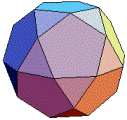HOME MATH DICTIONARY DOWNLOAD FEEDBACK DISCLAIMER
 Question: What is Polyhedron ? Answer: A polyhedron is a solid shape whose faces are all polygons. Polyhedra occur naturally as the shapes of crystals, for example, alum, copper sulphate and common salt. A face is bounded by a circuit of edges, and is usually a flat (plane) region called a polygon. The faces together make up the polyhedral surface. A polyhedron is a 3-dimensional example of the more general polytope in any number of dimensions. Example of a Polyhedron :